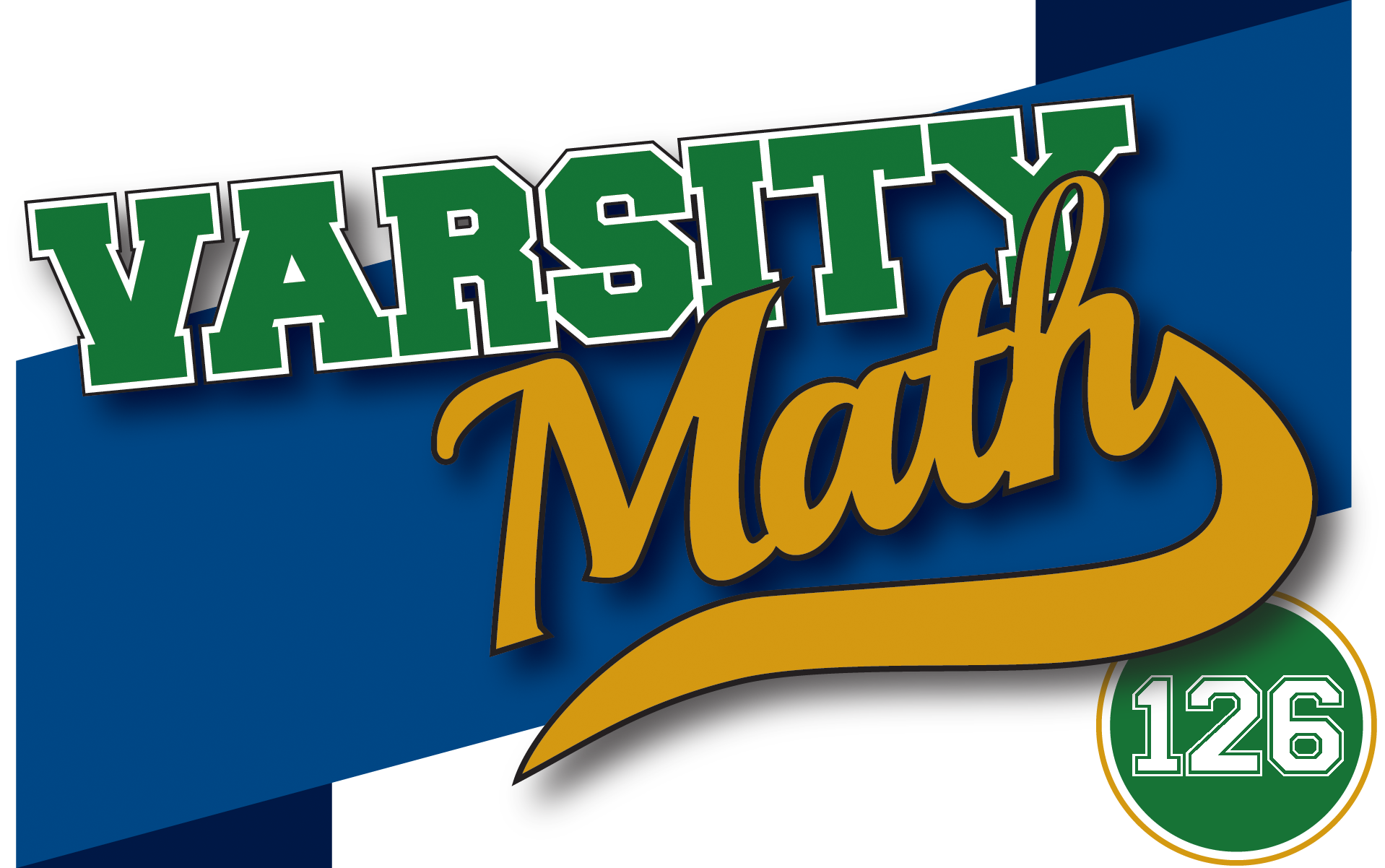## ________________

Some logical reasoning puzzles keep the team sharp.

## ________________### Logical Coffee Order

Four of the math team members known for their impeccable logic have finished dinner together. The server arrives and asks, “Do any of you want coffee?” The team members, not knowing in advance anyone’s preference for coffee, answer in turn:
Richard: “I don’t know.”
Cindy: “I don’t know.”
David: “I don’t know.”
Kayla: “Yes.”

Who should be served coffee and why?

### Here’s Looking at You

Adam is looking at Zoe.
Zoe is looking at Joshua.
Joshua is not married.

Is a married person looking at a person who is not married?
a. Yes
b. No
c. Not enough information

## Solutions to week 125

In Piece of Pi, “National Museum of Mathematics” is encoded in the decimal expansion of pi every 6.93×1059 digits or so. The solutions to Making 17 and 27 are 17 = 1 + .5-4 and 27 = 91.5.

Piece of Pi answer explained:
The expression “National Museum of Mathematics” contains 30 characters, which will require 60 digits to encode. A string of n digits of pi will provide n – 59 opportunities for 60 digits to match in consecutive order. For any one of these 60-digit strings the probability of making a hit is p = 10-60. The probability of missing on all n – 59 opportunities is Q = (1-p)n – 59. The natural logarithm of Q is lnQ = (n – 59)ln(1 – p) ≈ -np, so that Q ≈ e-np. If we set Q = .5, then n ≈ .69314/p = 6.93141059. Thus, “National Museum of Mathematics” is encoded in the decimal expansion of pi every 6.93×1059 digits or so.

Making 17 and 27 answer explained:
17 = 1 + .5-4 = 1 + 1/.54 = 1 + 24 = 1 + 16 = 17
27 = 91.5 = (32)1.5 = 33 = 27

## Recent Weeks

Links to all of the puzzles and solutions are on the Complete Varsity Math page.

Come back next week for answers and more puzzles.